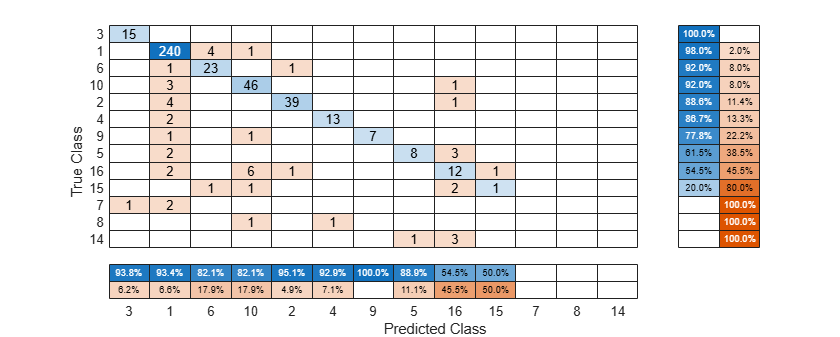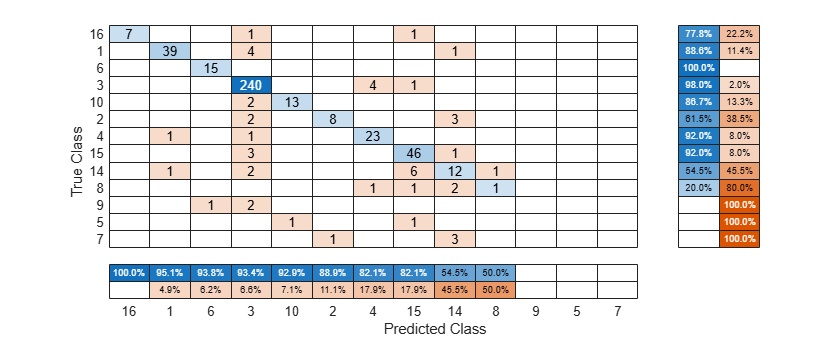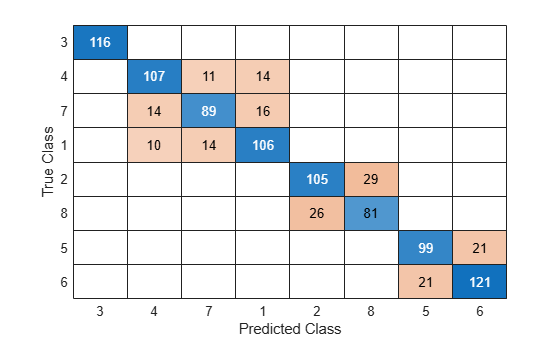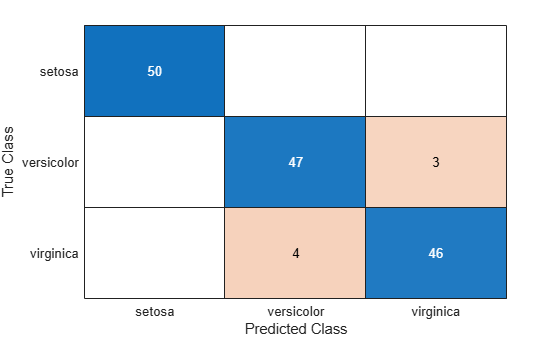# sortClasses

Sort classes of confusion matrix chart

## Syntax

``sortClasses(cm,order)``

## Description

example

``sortClasses(cm,order)` sorts the classes of the confusion matrix chart `cm` in the order specified by `order`. You can sort the classes in their natural order, by the values along the diagonal of the confusion matrix, or in fixed order that you specify.`

## Examples

collapse all

Create a confusion matrix chart and sort the classes of the chart according to the class-wise true positive rate (recall) or the class-wise positive predictive value (precision).

Load and inspect the `arrhythmia` data set.

```load arrhythmia isLabels = unique(Y); nLabels = numel(isLabels)```
```nLabels = 13 ```
`tabulate(categorical(Y))`
``` Value Count Percent 1 245 54.20% 2 44 9.73% 3 15 3.32% 4 15 3.32% 5 13 2.88% 6 25 5.53% 7 3 0.66% 8 2 0.44% 9 9 1.99% 10 50 11.06% 14 4 0.88% 15 5 1.11% 16 22 4.87% ```

The data contains 16 distinct labels that describe various degrees of arrhythmia, but the response (`Y`) includes only 13 distinct labels.

Train a classification tree and predict the resubstitution response of the tree.

```Mdl = fitctree(X,Y); predictedY = resubPredict(Mdl);```

Create a confusion matrix chart from the true labels `Y` and the predicted labels `predictedY`. Specify `'RowSummary'` as `'row-normalized'` to display the true positive rates and false positive rates in the row summary. Also, specify `'ColumnSummary'` as `'column-normalized'` to display the positive predictive values and false discovery rates in the column summary.

```fig = figure; cm = confusionchart(Y,predictedY,'RowSummary','row-normalized','ColumnSummary','column-normalized');```

Resize the container of the confusion chart so percentages appear in the row summary.

```fig_Position = fig.Position; fig_Position(3) = fig_Position(3)*1.5; fig.Position = fig_Position;```To sort the confusion matrix according to the true positive rate, normalize the cell values across each row by setting the `Normalization` property to `'row-normalized'` and then use `sortClasses`. After sorting, reset the `Normalization` property back to `'absolute'` to display the total number of observations in each cell.

```cm.Normalization = 'row-normalized'; sortClasses(cm,'descending-diagonal') cm.Normalization = 'absolute'; ```To sort the confusion matrix according to the positive predictive value, normalize the cell values across each column by setting the `Normalization` property to `'column-normalized'` and then use `sortClasses`. After sorting, reset the `Normalization` property back to `'absolute'` to display the total number of observations in each cell.

```cm.Normalization = 'column-normalized'; sortClasses(cm,'descending-diagonal') cm.Normalization = 'absolute'; ```Create a confusion matrix chart by using the `confusionchart` function, and sort the classes to cluster similar classes by using the `'cluster'` option of the `sortClasses` function. This example also shows how to cluster by using the `pdist`, `linkage`, and `optimalleaforder` functions.

Generate a sample data set that contains eight distinct classes.

```rng('default') % For reproducibility trueLabels = randi(8,1000,1); predictedLabels = trueLabels;```

Insert confusion among classes {1,4,7}, {2,8}, and {5,6} for the first 200 samples.

```rename = [4 8 3 7 6 5 1 2]; predictedLabels(1:100) = rename(predictedLabels(1:100)); rename = [7 8 3 1 6 5 4 2]; predictedLabels(101:200) = rename(predictedLabels(101:200));```

Create a confusion matrix chart from the true labels `trueLabels` and the predicted labels `predictedLabels`.

```figure cm1 = confusionchart(trueLabels,predictedLabels);```Cluster Using `'cluster'`

Sort the classes to cluster similar classes by using the `'cluster'` option.

`sortClasses(cm1,'cluster')`Cluster Using `pdist`, `linkage`, and `optimalleaforder`

Instead of using the `'cluster'` option, you can use the `pdist`, `linkage`, and `optimalleaforder` functions to cluster confusion matrix values. You can customize clustering by using the options of these functions. For details, see the corresponding function reference pages.

Suppose you have a confusion matrix and class labels.

```m = confusionmat(trueLabels,predictedLabels); labels = [1 2 3 4 5 6 7 8];```

Compute the clustered matrix and find the corresponding class labels by using `pdist`, `linkage`, and `optimalleaforder`. The `pdist` function computes the Euclidean distance `D` between pairs of the confusion matrix values. The `optimalleaforder` function returns an optimal leaf ordering for the hierarchical binary cluster tree `linkage(D)` using the distance `D`.

```D = pdist(m); idx = optimalleaforder(linkage(D),D); clusteredM = m(idx,idx); clusteredLabels = labels(idx);```

Create a confusion matrix chart using the clustered matrix and the corresponding class labels. Then, sort the classes using the class labels.

```cm2 = confusionchart(clusteredM,clusteredLabels); sortClasses(cm2,clusteredLabels)```The sorted confusion matrix chart `cm2`, which you created by using `pdist`, `linkage`, and `optimalleaforder`, is identical to the sorted confusion matrix chart `cm1`, which you created by using the `'cluster'` option.

Create a confusion matrix chart and sort the classes of the chart in a fixed order.

```load fisheriris X = meas([51:150,1:50],:); Y = species([51:150,1:50],:);```

`X` is a numeric matrix that contains four petal measurements for 150 irises. `Y` is a cell array of character vectors that contains the corresponding iris species.

Train a k-nearest neighbor (KNN) classifier, where the number of nearest neighbors in the predictors (k) is 5. A good practice is to standardize numeric predictor data.

`Mdl = fitcknn(X,Y,'NumNeighbors',5,'Standardize',1);`

Predict the labels of the training data.

`predictedY = resubPredict(Mdl);`

Create a confusion matrix chart from the true labels `Y` and the predicted labels `predictedY`.

`cm = confusionchart(Y,predictedY);`By default, `confusionchart` sorts the classes into their natural order as defined by `sort`. In this example, the class labels are character vectors, so `confusionchart` sorts the classes alphabetically. Reorder the classes of the confusion matrix chart in a fixed order.

`sortClasses(cm,["versicolor","setosa","virginica"])`## Input Arguments

collapse all

Confusion matrix chart, specified as a `ConfusionMatrixChart` object. To create a confusion matrix chart, use `confusionchart`,

Order in which to sort the classes of the confusion matrix chart, specified as one of these values:

• `'auto'` — Sorts the classes into their natural order as defined by the `sort` function. For example, if the class labels of the confusion matrix chart are a string vector, then sort alphabetically. If the class labels are an ordinal categorical vector, then use the order of the class labels.

• `'ascending-diagonal'` — Sort the classes so that the values along the diagonal of the confusion matrix increase from top left to bottom right.

• `'descending-diagonal'` — Sort the classes so that the values along the diagonal of the confusion matrix decrease from top left to bottom right.

• `'cluster'` — Sort the classes to cluster similar classes. You can customize clustering by using the `pdist`, `linkage`, and `optimalleaforder` functions. For details, see Sort Classes to Cluster Similar Classes.

• Array — Sort the classes in a unique order specified by a categorical vector, numeric vector, string vector, character array, cell array of character vectors, or logical vector. The array must be a permutation of the `ClassLabels` property of the confusion matrix chart.

Example: `sortClasses(cm,'ascending-diagonal')`

Example: `sortClasses(cm,["owl","cat","toad"])`

### Properties

Introduced in R2018b